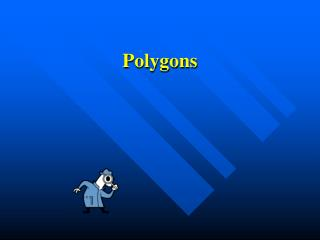DownloadDownload PresentationPolygons

# Polygons

Download Presentation## Polygons

- - - - - - - - - - - - - - - - - - - - - - - - - - - E N D - - - - - - - - - - - - - - - - - - - - - - - - - - -
##### Presentation Transcript

1. Polygons

2. Polygons • Simple, • Closed figure • Made up of line segments

3. Types of Polygons Triangles three sides Quadrilaterals four sides

4. Pentagon five sides

5. Pentagon five sides Hexagon six sides Heptagon seven sides

6. septuplets

7. Octagon eight sides

8. STOP

9. Octagon eight sides Nonagon nine sides Decagon ten sides

10. Review A polygon is a simple, closed, figure made up of line segments. A polygon is classified by the number of sides it has.

11. Can you classify these polygons?

12. Regular Polygons • A regular polygon has all sides and all angles congruent. B A Which is a regular polygon?

13. Triangles • Triangles can be classified by their sides or by their angles.

14. Triangles Classifying triangles by the angles….

15. Acute TriangleAll angles are acute.

16. Right TriangleContains one right angle.

17. Obtuse TriangleContains one obtuse angle.

18. Classify Triangles by their angles • Acute • All angles are acute • Right • Contains one right angle • Obtuse • Contains one obtuse angle

19. TRIANGLES Classifying triangles by their sides…

20. TRIANGLES SCALENE

21. TRIANGLES SCALENE ISOSCELES

22. TRIANGLES SCALENE ISOSCELES EQUILATERAL

23. Classify triangles by its sides • Scalene • No sides congruent • Isosceles • Two sides congruent • Equilateral • All sides congruent

24. WHAT IS A REGULAR TRIANGLE CALLED?